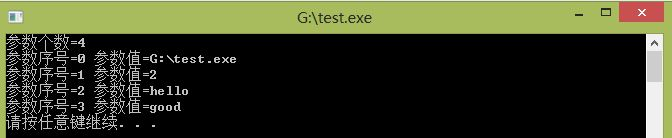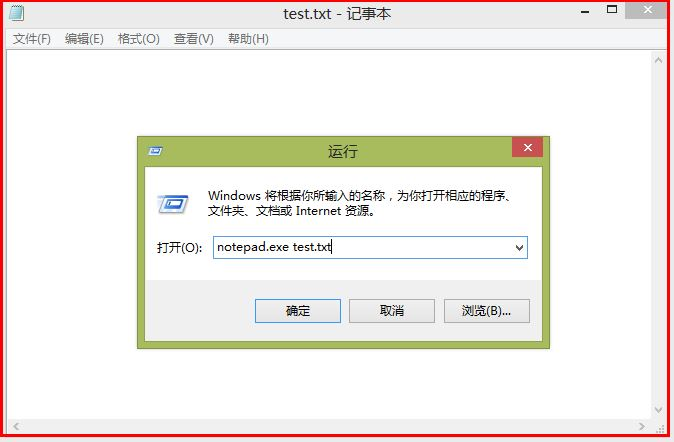# 【c语言学习笔记二十三】main函数的参数argc和argv

ANSI-C(美国国家标准协会,C的第一个标准ANSI发布)在C89/C99中main()函数主要形式为:
(1).int main(void)
(2).int main(int argc,char *argv[]) = int main(int argc,char **argv).

(参照
Arguments to main和C++ Primer7.2.6节)
(1).int argc:英文名为arguments count(参数计数)
count of cmd line args,运行程序传送给main函数的命令行参数总个数,包括可执行程序名,其中当argc=1时表示只有一个程序名称,此时存储在argv中.
(2).char **argv:英文名为arguments value/vector(参数值)
pointer to table of cmd line args,字符串数组,用来存放指向字符串参数的指针数组,每个元素指向一个参数,空格分隔参数,其长度为argc.数组下标从0开始,argv[argc]=NULL.
argv 指向程序运行时的全路径名
argv 指向程序在DOS命令中执行程序名后的第一个字符串
argv 指向执行程序名后的第二个字符串
argv[argc] 为NULL.

1.计算命令行参数个数程序如下:

//C 输出参数个数
#include <stdio.h>
#include <stdlib.h>
int main(int argc,char *argv[])
{
printf(“参数个数=%d\n”,argc);
system(“PAUSE”);
return 0;
}

//C++ 输出参数个数
#include <iostream>
using namespace std;
int main(int argc,char *argv[])
{
cout<<“参数个数=”<<argc<<endl;
system(“PAUSE”);
return 0;
}2.查看argv[]二维数组存储具体字符串的代码如下:

//C 查看argv存储参数值及对应序号
#include <stdio.h>
#include <stdlib.h>
int main(int argc,char *argv[])
{
int i;
printf(“参数个数=%d\n”,argc);
for(i=0; i<argc; i++)
{
printf(“参数序号=%d “,i);
printf(“参数值=%s\n”,argv[i]);
}
system(“PAUSE”);
return 0;
}

//C++ 查看argv存储参数值及对应序号
#include <iostream>
using namespace std;
int main(int argc,char *argv[])
{
cout<<“参数个数=”<<argc<<endl;
for(int i=0; i<argc; i++)
{
cout<<“参数序号=”<<i<<” “;
cout<<“参数值=”<<argv[i]<<endl;
}
system(“PAUSE”);
return 0;
}

“运行”中输入”G:\test.exe 2 hello good”,则输出入下图所示:#include <iostream>
using namespace std;
int main(int argc,char *argv[])
{
//参数个数=3:工程名\读取txt文件\写入txt文件
if(argc!=3)
throw runtime_error(“wrong number of arguments”);
//打开转换文件,argv为读取的要转换txt文件名 open_file自定义打开函数
if(!open_file(map_file,argv))
throw runtime_error(“no transformation file”);
//打开要转换的写入txt文件
if(!open_file(input,argv))
throw runtime_error(“no input file”);
return 0;
}